GFG App
Open AppBrowser
Continue

# Unweighted or Simple Index Numbers: Meaning and Methods

A statistical measure that helps in finding out the percentage change in the values of different variables, such as the price of different goods, production of different goods, etc., over time is known as the Index Number. The percentage change is determined by taking a base year as a reference. This base year is the year of comparison. When an investigator studies different goods simultaneously, then the percentage change is considered the average for all the goods. There are two broad categories of Index Numbers: viz., Simple or Unweighted and Weighted Index Numbers.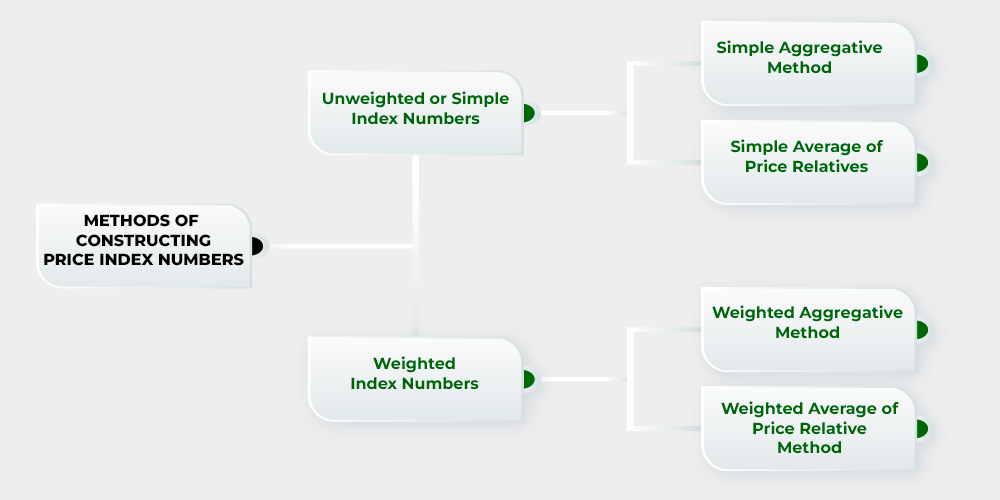### Unweighted or Simple Index Numbers

An Index Number in which each item must have some weight as no weight is expressly assigned to any item is known as Unweighted Index Number. It is also known as Simple Index Number and can be constructed with the help of two techniques; viz., Simple Aggregative Method and Simple Average of Price Relatives Method.

### 1. Simple Aggregative Method:

A method in which the aggregate prices of all the selected commodities in the current year are expressed as the aggregate price of all the commodities in the base year is known as Simple Aggregative Method. It is the simplest method to construct index number. The steps required to construct index number through this method are as follows:

1. The first step is to add up the current year prices of different commodities and denote the sum by ∑p1.
2. Now, the base year prices of the commodities are added up, and the sum is denoted by ∑p0.
3. The calculated values are now put in the following formula:Where,

P01 = Index Number of the Current Year

∑p1 = Total of the current year’s price of all commodities

∑p0 = Total of the base year’s price of all commodities

#### Example:

From the following data, construct Index Numbers for 2019-2020, taking 2012-2013 as the base year by using Simple Aggregate Method.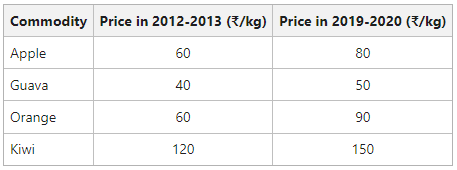#### Solution: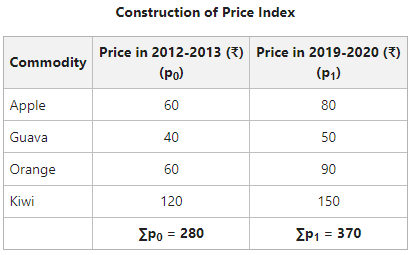Price Index for year 2019-2020 with year 2012-2013 as the base:The value of Price Index (132.14) reveals that in the year 2019-2020, there is a net increase of 32.14% in the prices of given commodities as compared to the year 2012-2013.

#### Limitations of Simple Aggregative Method:

1. Influenced by the magnitude of the prices: If the price of a commodity is higher, it will have a great influence on the index number. It means that the commodities with higher prices receive greater weightage than the commodities with lower prices. For example, in the above example price of Kiwi is higher than the price of Orange, Guava, and Apple; hence, Kiwi will influence the index number more than other fruits.

2. Equal Weights are assigned to every item: While constructing index, equal weights are assigned to every item and the relative importance of different commodities is not taken into account. For example, In this index, equal importance is given to eraser and milk.

3. Prices of various commodities may be quoted in different units: Another disadvantage of this method is that the price of various commodities may be shown in different units, such as rupees per kg, rupees per litre, rupees per quintal, etc. Therefore, with this method, the index is influenced by the quoted units of the commodities, which means that some commodities may get more importance as they are quoted in a specific unit.

### 2. Simple Average of Price Relatives Method:

This index number is an improvement over the previous method, as it is not affected by the unit in which the prices of the commodities are quoted. The steps required to construct index number through this method are as follows:

1. Firstly, calculate the price relatives of the current year; i.e., divide the price of each commodity in the current year by the price in the base year.
2. Then determine the sum total of price relatives; i.e.,3. Now, divide the sum total of the price relatives of every commodity by the number of commodities.
4. Use the following formula to determine Index Number:#### Example:

From the following data, construct an index for 2019-2020, taking 2012-2013 as the base by using Simple Average of Price Relatives Method.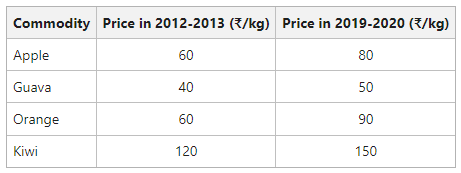#### Solution: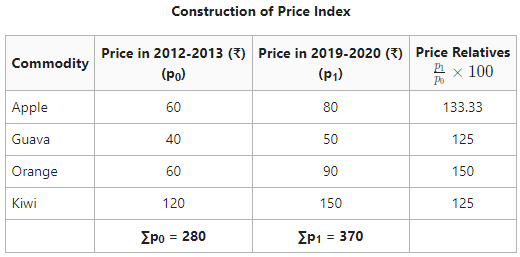The Price Index Number (133.33) shows that there is an increase of 33.33% in the prices of commodities in the year 2019-2020 as compared in the year 2012-2013.

### Merits of Simple Average of Price Relatives Method

• The value of the index by using the simple average of price relatives method is not affected by the units in which the price of the commodity is quoted. As pure relatives are pure numbers, they are independent of the original units in which they are quoted.
• In this method, each commodity gets equal importance, and the extreme conditions do not have any influence on the index number.

### Demerits of Simple Average of Price Relatives Method

• As this method is an unweighted index, equal importance is given to each price relative. However, in reality, few of the price relatives are more important than the others.
• One faces difficulty with this method while selecting an appropriate average.

My Personal Notes arrow_drop_up